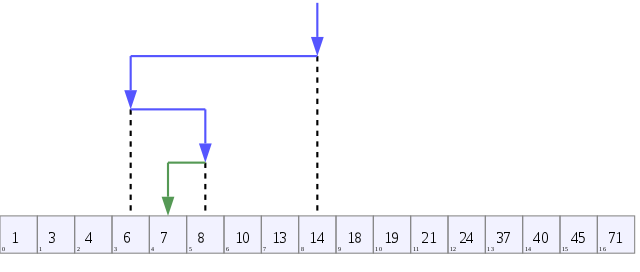by Pablo E. Cortez

# Binary Search Algorithms Explained using C++

Binary search is one of those algorithms that you’ll come across on every (good) introductory computer science class. It’s an efficient algorithm for finding an item in an ordered list. For the sake of this example we’ll just assume this is an array.

The goals of binary search is to:

• be able to discard half of the array at every iteration
• minimize the number of elements we have to go through
• leave us with one final value

Take for example the following array of integers:

``int array[] = {     1, 3, 4, 6, 7, 8, 10, 13, 14, 18, 19, 21, 24, 37, 40, 45, 71 };``

Let’s say we are trying to find the index value of the number 7 in this array. There are 17 items in total and the index values go from 0 to 16.

We can see that the index value of 7 is 4, since it’s the fifth element in the array.

But what would be the best way for the computer to find the index value of the number we are looking for?

First, we store the `min` and `max` values, such as `0` and `16`.

``int min = 0;int max = 16;``

Now we have to come up with a guess. The smartest thing to do would be to guess an index value in the middle of the array.

With the index value 0 to 16 in this array, the middle index value of this array would be 8. That holds the number 14.

`// This will round down if the quotient is not an integer`
`int guess = (min + max) / 2;`

Our guess is now equal to 8, which is 14 in the array, since `array` is equal to `14` .(Wikimedia Commons.) The first guess is at index value 8, which stores the number 14.

If the number we were looking for was 14, we would be done!

Since that is not the case, we will now discard half of the array. These are all the numbers after 14, or index value 8, since we know that 14 is greater than 7, and our guess is too high.

After the first iteration, our search is now within: `1, 3, 4, 6, 7, 8, 10, 13`

We don’t have to guess in the last half of the original array, because we know that all those values are too big. That’s why it’s important that we apply binary search to an ordered list.

Since our original guess of 14 was greater than 7, we now decrease it by 1 and store that into `max`:

``max = guess - 1; // max is now equal to 7, which is 13 in the array``

Now the search looks like this:

``                      1, 3, 4, 6, 7, 8, 10, 13``
``min = 0max = 7guess = 3 ``

Because our guess was too low, we discard the bottom half of the array by increasing the `min`, conversely to what we previously did to `max`:

``min = guess + 1; // min is now 4``

By the next iteration, we are left with:

``                             7, 8, 10, 13min = 4max = 7guess = 5``

Since index value 5 returns 8, we are now one over our target. We repeat the process again, and we are left with:

``                                  7min = 4max = 4guess = 4``

And we are left with only one value, 4, as the index of the target number we were looking for, which was 7.

The purpose of binary search is to get rid of half of the array at every iteration. So we only work on those values on which it makes sense to keep guessing.

The pseudo-code for this algorithm would look something like this:

1. Let `min = 0` , and let `max = n` where `n` is the highest possible index value
2. Find the average of `min` and `max` , round down so it’s an integer. This is our `guess`
3. If we guessed the number, stop, we got it!
4. If `guess`is too low, set `min` equal to one more than `guess`
5. If `guess`is too high, set `max` equal to one less than `guess`
6. Go back to step two.

Here’s a solution, written in C++: